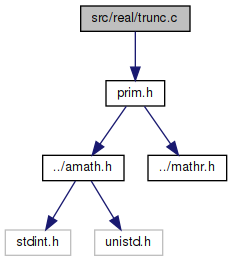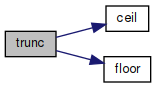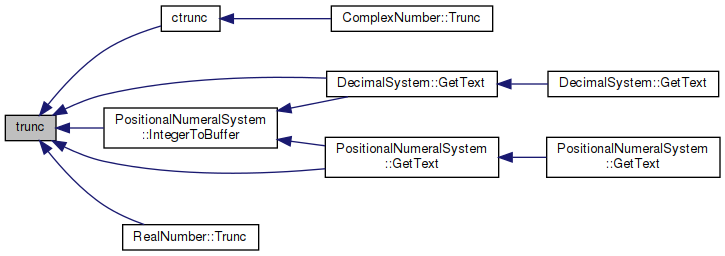amath  1.8.5 Simple command line calculator
trunc.c File Reference

Truncate function. More...

`#include "prim.h"`
Include dependency graph for trunc.c:Go to the source code of this file.

## Functions

double trunc (double x)
Truncate function. More...

## Detailed Description

Truncate function.

Definition in file trunc.c.

## ◆ trunc()

 double trunc ( double x )

Truncate function.

```when x > 0
trunc(0)   = floor(x)```
```when x < 0
trunc(x)   = ceil(x)```
```Special case
trunc(0)   = 0
trunc(NaN) = NaN
```

Definition at line 52 of file trunc.c.

References ceil(), and floor().

53 {
54  uint32_t hx, lx;
55  GET_HIGH_WORD(hx, x);
56  if ((hx & 0x7FF00000) == 0x7FF00000)
57  {
58  return NAN;
59  }
60
61  GET_LOW_WORD(lx, x);
62  if (hx == 0 && lx == 0)
63  {
64  return 0.0;
65  }
66
67  if ((hx & 0x80000000) != 0x80000000)
68  {
69  return floor(x);
70  }
71
72  return ceil(x);
73 }
#define GET_HIGH_WORD(i, d)
Get the more significant 32 bit int from a double.
Definition: prim.h:167
double ceil(double x)
Ceiling function.
Definition: ceil.c:63
#define GET_LOW_WORD(i, d)
Get the less significant 32 bit int from a double.
Definition: prim.h:177
#define NAN
Definition: mathr.h:53
double floor(double x)
Floor function.
Definition: floor.c:62
Here is the call graph for this function:Here is the caller graph for this function: